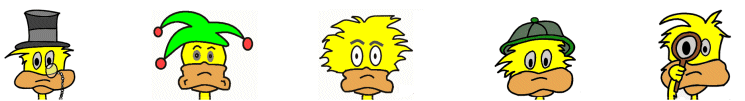# Kids Math

## Glossary and Terms: Fractions

Complex fraction - A complex fraction is a fraction where the numerator and/or denominator are a fraction.

Decimal - A decimal is a number based on the number 10. It can be thought of as a special type of fraction where the denominator is a power of 10.

Decimal point - A period or dot that is part of a decimal number. It indicates where the whole number stops and the fraction portion begins.

Denominator - The bottom part of a fraction. It shows how many equal parts that the item has been divided into.

Example: In the fraction 3/4 , 4 is the denominator

Equivalent fractions - These are fractions that may look different, but have the same value.

Example: ¼ = 2/8 = 25/100

Fraction - A part of a whole. A common fraction is made up of a numerator and a denominator. The numerator is shown on top of a line and is the number of parts of the whole. The denominator is shown below the line and is the number of parts by which the whole has been divided.

Example: 2/3, in this fraction the whole has been divided into three parts. This fraction represents 2 parts of the 3.

Half - Half is a common fraction that can be written ½. It can also be written as .5 or 50%.

Higher term fraction - A higher term fraction means that the numerator and denominator of the fraction have a factor in common other than one. In other words, the fraction could be reduced further.

Example: 2/8; this is a higher term fraction because both 2 and 8 have the factor 2 and 2/8 can be reduced to 1/4.

Improper fraction - A fraction where the numerator is greater than the denominator. It has a value greater than 1.

Example: 5/4

Lowest term fraction - A fraction that has been fully reduced. The only common factor between the numerator and denominator is 1.

Example: 3/4 , this is a lowest term fraction. It cannot be further reduced.

Mixed number - A number that is made up of a whole number plus a fraction.

Example: 3 1/4

Numerator - The top part of a fraction. It shows how many equal parts of the denominator are represented.

Example: In the fraction 3/4 , 3 is the numerator

Percent - A percent is a special type of fraction where the denominator is 100. It can be written using the % sign.

Example: 50%, this is the same as ½ or 50/100

Proper fraction - A proper fraction is a fraction where the numerator (the top number) is less that the denominator (the bottom number).

Example: ¾ and 7/8 are proper fractions

Proportion - An equation stating that two ratios are equivalent is called a proportion.

Example: 1/3 = 2/6 is a proportion

Ratio - A ratio is a comparison of two numbers. It can be written a few different ways.

Example: The following are all ways to write the same ratio: 1/2 , 1:2, 1 of 2

Reciprocal - The reciprocal of a fraction is when the numerator and denominator are switched. When you multiply the reciprocal with the original number, you always get the number 1. All numbers have a reciprocal except for 0.

Example: The reciprocal of 3/8 is 8/3. The reciprocal of 4 is ¼.

More Math Glossaries and Terms

Algebra glossary
Angles glossary
Figures and Shapes glossary
Fractions glossary
Graphs and lines glossary
Measurements glossary
Mathematical operations glossary
Probability and statistics glossary
Types of numbers glossary
Units of measurements glossary

Back to Kids Math

Back to Kids Study